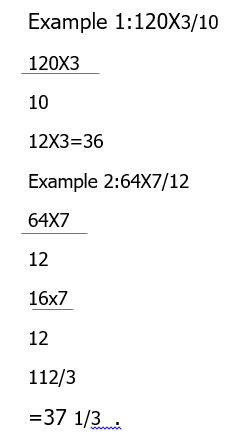### TOPIC: Multiplication and Division of Fraction.

Mathematics Jss1 first Term.

Week 10.

TOPIC: Multiplication and Division of Fraction.

Performance Objectives:

Students should be able to

1. Solve problems on multiplication of fractions.
2. Solve problems on Division of fractions.
3. Solve word problems on multiplication of fractions.

Content.5.6x2 3/4x2 2/11         A) 36       B) 34         C)32          D)28.

PERIOD 2.

Example 1:Simplify 2 1/5÷1/4

11/5 ÷1/4

11/5x4/1

44/5

8 4/5 .

Note in division of fraction, the sign(÷) changes after a fraction to (X).

Example 2:1 5/9÷3 4/9

14/9÷31/9

14/9x9/31

=14/31.

View More